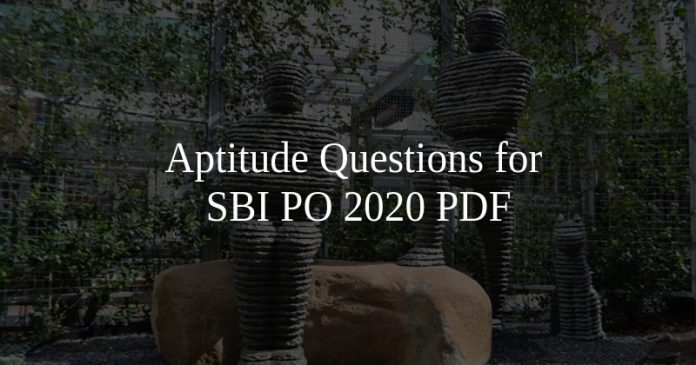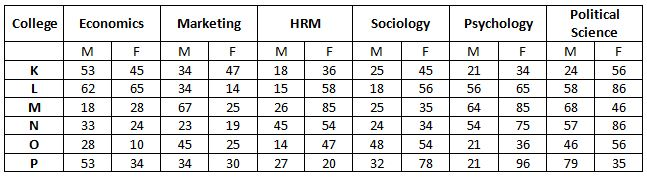# Aptitude Questions for SBI PO 2020 PDF

0
346Aptitude Questions for SBI PO 2020 PDF

# Aptitude Questions for SBI PO 2020 PDF

Download SBI PO Aptitude Questions & Answers PDF for SBI PO Prelims and Mains exam. Top-15 Very Important SBI PO Questions with solutions for Banking Exams.

Instructions

What will come in place of the question mark (?) in the following questions ?

Question 1: $\frac{3}{8}$ of $((4624 \div (564-428))=?$

a) $13\frac{1}{4}$

b) $14\frac{1}{2}$

c) $11\frac{5}{8}$

d) $12\frac{3}{4}$

e) $12\frac{1}{8}$

Question 2: $456\div24\times38-958\div364\times182 = ?$

a) 112

b) 154

c) 128

d) 243

e) 118

Question 3: Ms. Sujata invests 7% i.e. Rs. 2170/- of her monthly salary in mutual funds. Later she invests 18% of her monthly salary in recurring deposits also, she invests 6% of her salary on NSC’s. What is the total annual amount invested by Ms. Sujata ?

a) Rs. 1, 25, 320/-

b) Rs. 1, 13, 520/-

c) Rs. 1, 35, 120/-

d) Rs. 1, 15, 320/-

e) None of these

Question 4: What is the 30% of 40% of $\frac{2}{5}$th of 2500 ?

a) 500

b) 400

c) 360

d) 120

e) None of these

Question 5: 2 women and 10 children together take 8 days to complete a piece of work How many days will 10 children alone take to complete the piece of work if 8 women alone can complete the piece of work in 6 days ?

a) 15

b) 12

c) 10

d) 24

e) None of these

SBI PO Free Mock Tests

Question 6: 10 women can complete a work in 8 days and 10 children take 12 days to complete the work. How many days will 6 women and 3 children together take to complete the work?

a) 9

b) 12

c) 7

d) 8

e) None of these

Question 7: 960, 839, 758, 709, ?, 675

a) 696

b) 700

c) 688

d) 678

e) 684

Question 8: 61, 72, ?, 73, 59, 74, 58

a) 70

b) 60

c) 71

d) 62

e) 63

Question 9: If 6 boys and 6 girls have to sit in a round circular music chair. So, that there is a girl between every 2 boys. Find the number of ways they can sit?

a) 6! × 5!

b) 6! × 4!

c) 6! × 3!

d) 6! × 2!

e) None of these

Question 10: What is the number of words formed from the letters of the word ‘JOKE’ So that the vowels and consonants alternate?

a) 4

b) 8

c) 12

d) 18

e) None of these

Question 11: There are two motor cycles’ (A and B) of equal cost price. Motorcycle A was sold at a profit of 14% and motorcycle B was sold for Rs.4,290/­ more than its cost price. The net profit earned after selling both the motor cycles (A and B) is 20%. What is the cost price of each motorcycle ?

a) Rs. 16,500/­

b) Rs. 16,000/­

c) Rs. 15,500/­

d) Rs. 17,500/­

e) Rs. 17,000/­

Question 12: A shopkeeper purchased 15 kg of variety A rice at X per kg and 10 kg of variety B rice at ‘X+ 5’ per kg. The shopkeeper sold the whole quantity of variety A rice at 10% profit and that of variety B rice at 20% profit. The total selling price of variety A rice was 30/- more than that of variety B rice. Had the two varieties been mixed and sold at an overall profit of 20%, what would have been the selling price of the mixture per kg?

a) Rs. 26.40

b) Rs. 23.20

c) Rs. 24.20

d) Rs. 25.00

e) Rs. 28.30

Instructions

Study the following table and answer the questions that follow:Question 13: The total number of females specializing in Political Science from college K,N and P together are approximately what percent of the males
specializing in the same field from the same colleges ?

a) 210

b) 90

c) 190

d) 150

e) 110

Question 14: How many students are there in college M from all the specializations together ?

a) 574

b) 576

c) 572

d) 568

e) None of these

Question 15: The radius of a circular field is equal to the side of a square field whose perimeter is 784 feet. What is the area of the circular field?

a) 107914 sq ft

b) 120736 sq ft

c) 107362 sq ft

d) 127306 sq ft

e) None of these

Answers & Solutions:

1) Answer (D)

$\frac{3}{8}$ of $((4624 \div (564-428))=?$

$\frac{3}{8}$ of $((4624 \div (136))=?$

$\frac{3}{8}$ of $(34)=?$

$\frac{51}{4}$

12$\frac{3}{4}$

2) Answer (D)

? =456 ÷ 24 × 38 – 958 ÷ 364 × 182

? =19 × 38 – 958 ÷ 2

? =19 × 38 – 479

? = 722 – 479

? = 243

3) Answer (D)

The total percentage of her salary that Sujata invested is 7% + 18% + 6% = 31%

7% of her salary is Rs. 2170.
So, 31% of her salary is 2170/7 * 31 = 9610

So, Sujata invests Rs. 9610 every month i.e 9610*12 = 115320

4) Answer (D)

$\frac{2}{5} \times 2500 = 1000$
So, 40% of 1000 = 400
30% of 400 = 120

5) Answer (B)

Let the speed of women be x and that of children be y. Let the piece of work be W.
2x + 10y = W/8
8x = W/6
x = W/48
Hence, we get y= W/120
Speed of 1 child = W/120
Speed of 10 children = W/12
Now time needed for them to complete = W/(W/12) =12days
Therefore, the correct option is B.

6) Answer (E)

10 women can complete the work in 8 days. So, number of hours required for 1 woman to complete the work = 80

Number of hours required by one child to complete the work = 120

So, 80W = 120C => 1W = 1.5C

6 women + 3 children = 9 children + 3 children = 12 children

So, 12 children take 10 days to complete the work.

7) Answer (E)

$960 – 11^2 = 839$

$839 – 9^2 = 758$

$758 – 7^2 = 709$

$709 – 5^2 = 684$

$684 – 3^2 = 675$

Hence, the missing number is 684

8) Answer (B)

For first, third, fifth and seventh number:

61-1 = 60

60-1 = 59

59-1 = 58

For fourth and sixth number:

72+1 = 73

73+1 = 74

Hence, answer will be B

9) Answer (A)

Circular permutation = n! (n – 1)!

∴ Number of ways = 6! (6 – 1)! = 6! × 5!

10) Answer (B)

Word name: ‘JOKE’ Vowels: O, E
Consonants: J, K
∴ Possible arrangement beginning with consonant: JOKE, KOJE, JEKO, KEJO = 4 Numbers
beginning with vowel: OJEK, OKEJ, EJOK, EKOJ = 4 Numbers
Required number = 4+4 = 8 numbers

11) Answer (A)

Cost price of both A and B be X.
SP of A = 1.14 CP of A
SP of B = CP of B + 4290
Net profit after selling both bikes is 20%
1.2 (X+X) = SP of A +SP of B
1.2 (X+X) = 1.14 CP of A + CP of B + 4290 = 1.14X + X + 4290
2.4X = 2.14 X + 4290
0.26 X =4290
X = 16,500
Option A is the correct answer.

12) Answer (A)

Total revenue generated by selling variety A rice = 1.1*15x = 16.50x
Total revenue generated by selling variety B rice = 1.2*10(x+5) = 12x + 60

It is given that, 16.5x – 12x – 60 = 30
$\Rightarrow$ x = 20.

When the two varieties been mixed and sold at an overall profit of 20%. Total weight of the entire mixture = 10+15 = 25 kg.
Total cost price of the mixture = 15*20 + 10*25 = 550
Therefore, the cost price pf the mixture per kg = \dfrac{550}{25} = Rs. 22 per kg.
It is known that the mixture was sold at 20%. Therefore, the selling price of the mixture = 1.2*22 = Rs. 26.40.
Hence, option A is the correct answer.

13) Answer (E)

The required percent = (Number of females/Number of males)*10 = (57+79+24)/(56+86+35)*100=(177/166)*100=110

14) Answer (C)

Total number of student is addition of all the students doing all the specialization = 18+28+67+25+26+85+25+35+64+85= 572

15) Answer (B)

Perimeter of square field = 784 ft.

Radius of circular field = Side of square field = $\frac{784}{4}$ = 196 ft.

Area of circular field = $\pi r^2 = \frac{22}{7}\times196\times196 = 120736$ sq ft

We hope this Aptitude Questions & Answers  PDF for SBI PO Exams preparation is so helpful to you.